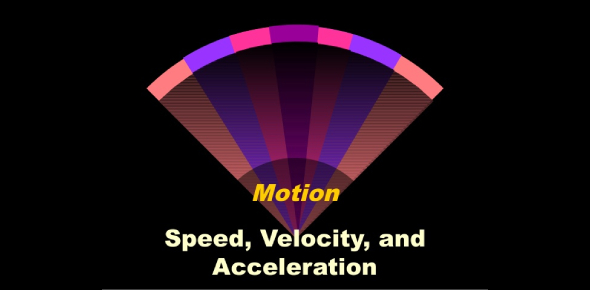# 8th Grade Science Quiz On Motion! Trivia

8 Questions | Total Attempts: 1324Settings.

• 1.
_________ occurs when the velocity of an object changes.
• A.

Acceleration

• B.

Velocity

• C.

Speed

• D.

Distance

• 2.
When you say that a race car travels northward at 100 km/h, you are talking about its _________
• A.

Velocity

• B.

Distance

• C.

Acceleration

• D.

Speed

• 3.
If a train is slowing down, it is accelerating
• A.

True

• B.

False

• 4.
What is the distance a measure of?
• A.

Width

• B.

Length

• C.

Hieght

• D.

Area

• 5.
What unit is the distance measured in?
• A.

Meters

• B.

Grams

• C.

Inches

• D.

Quarts

• 6.
Speed in a given direction is...
• 7.
The basic SI unit of length is the...
• 8.
Scientists around the world use the ________, a system of measurements based on the number ten.
Related TopicsBack to top